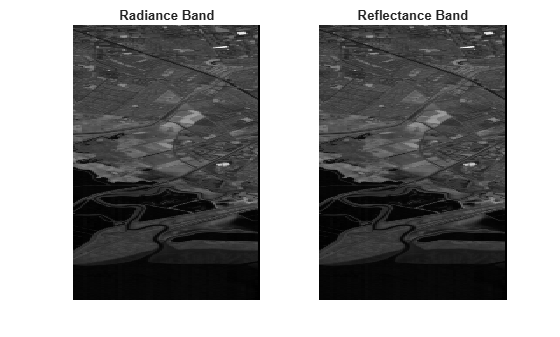Description

example

newhcube = radiance2Reflectance(hcube) converts the pixel values of the hyperspectral data cube from radiance to reflectance values. The function returns a new hypercube object and the pixel values of the data cube represent the top of atmosphere (TOA) reflectance. For more information, see TOA Reflectance.

newhcube = radiance2Reflectance(hcube,'BlockSize',blocksize) specifies the block size for block processing of the hyperspectral data cube by using the name-value pair argument 'BlockSize'.

The function divides the input image into distinct blocks, processes each block, and then concatenates the processed output of each block to form the output matrix. Hyperspectral images are multi-dimensional data sets that can be too large to fit in system memory in their entirety. This can cause the system to run out of memory while running the radiance2Reflectance function. If you encounter such an issue, perform block processing by using this syntax.

For example, radiance2Reflectance(hcube,'BlockSize',[50 50]) divides the input image into non-overlapping blocks of size 50-by-50 and then computes the reflectance values for pixels in each block.

Note

To perform block processing by specifying the 'BlockSize' name-value pair argument, you must have MATLAB® R2021a or a later release.

Note

This function requires the Image Processing Toolbox™ Hyperspectral Imaging Library. You can install the Image Processing Toolbox Hyperspectral Imaging Library from Add-On Explorer. For more information about installing add-ons, see Get and Manage Add-Ons.

Examples

collapse all

Read hyperspectral data into the workspace.

input = hypercube('EO1H0440342002212110PY_cropped.hdr');

Remove the bad spectral bands from the data cube.

input = removeBands(input,'BandNumber',bandNumber);

Convert the digital numbers to radiance values by using the dn2radiance function.

Convert the radiance values to reflectance values by using the radiance2Reflectance function.

Read and display the 80th spectral band in the input radiance and the output reflectance data.

reflectanceBand = newhcube.DataCube;
band = 80;

figure
subplot(1,2,1)
axis off
subplot(1,2,2)
imagesc(reflectanceBand(:,:,band))
title('Reflectance Band')

colormap(gray)
axis offInput Arguments

collapse all

Input hyperspectral data, specified as a hypercube object. The DataCube property of the hypercube object stores the hyperspectral data cube. The pixels values of the data cube must be radiance values specifying the amount of radiation from the surface being imaged. You can convert the pixel values in digital numbers to radiance values by using dn2radiance function.

Size of the data blocks, specified as a 2-element vector of positive integers. The elements of the vector correspond to the number of rows and columns in each block, respectively. The size of the data blocks must be less than the size of the input image. Dividing the hyperspectral images into smaller blocks enables you process large data sets without running out of memory.

• If the blocksize value is too small, the memory usage of the function reduces at the cost of increased execution time.

• If the blocksize value is large or equal to the input image size, the execution time reduces at the cost of increased memory usage.

Example: 'BlockSize',[20 20] specifies the size of each data block as 20-by-20.

Output Arguments

collapse all

Output hyperspectral data, returned as a hypercube object. The pixels values in the output data cube are top of atmosphere (TOA) reflectance values.

collapse all

TOA Reflectance

The TOA reflectance values specifies the ratio of radiation reflected by the surface to the radiation incident on the surface.

d is the earth-sun distance in astronomical units, ESUNλ is the mean solar irradiance for each spectral band, and θE is the sun elevation angle.

Lλ is the spectral radiance computed as:

Gain and Bias are the gain and offset values for each spectral bands respectively. The Metadata property of hypercube object contains the gain and offset values.